V.5 No 2 5 Supplement 1The point, how Einstein crushed down his second postulate - constant speed of light in all inertial frames - was enlighted in details in the item 2 of our paper. With this postulate, the constancy of 4-metric in transition to the space with gravity fields is also crushed down. Einstein clearly indicated it in his works: “The theory of relativity formally follows from the premise that for each allowed transformation of space-time variables, the expression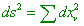(13)
 is invariant; this premise follows from the principle of constant speed of light. In accordance with it, only linear orthogonal transformations are solvable. The equation of freely moving material point in the Hamilton’s form is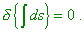(14)
 But in case if we refuse the postulate of constant speed of light, there will exist no coordinate systems selected a priori. So the coordinates xcan be meanwhile substituted by absolutely arbitrary functions of these values. But if there exists the four-dimensional regions in which, with the according choice of coordinates x, the material point moves as (13) and (14), we already may not consider them as an uniform law of motion of point in absence of forces. Instead, we have to add to (14) an equality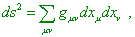(15)
 where the values g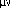are the functions of x” [18, p. 320]. “Thus, we come to persuasion that in general case, the gravity field is characterised by ten space-time functions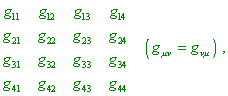(16)
 which in case of usual theory of relativity (SR - Authors) are equal, accordingly, to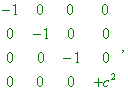(17)
 where c is a constant. The degeneration of the same kind takes place in a static gravity field of the considered type, with the difference that in that case g44 = c2 is the function of x1, x2, x3” [17, p. 231]. At the same time, the pass from Minkowski metric (13) to the generalised metric is not so simple. Even in case of static gravity field, it is not limited by the dependence of fourth tensor on spatial coordinates. “We can conclude from the above that between the space-time coordinates x1, x2, x3, x4 and the result of measurement obtained with help of scales and clocks there does not exist such simple relationship as in usual (SR - Authors) theory of relativity. With reference to time, this was revealed already for the stationary gravity field” [19, p. 233]. “Namely in order, the principle of constant speed of light to be true, the equation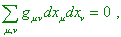(18)
 which determines the light propagation has to pass to the equation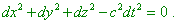It follows from this, with some choice of reference frame, the relation(19)
 has to be true, where now we made the substitution x1 = x, x2 = y, x3 = z, x4 = ct.. Consequently, the system of values gis degenerated into the system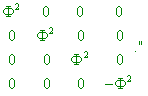(20)
 [20, p. 308]. It is very easy for us to check it with Schwarzschild metric, which is known to be yielded namely for stationary gravity field of a point source. In the natural coordinates x1, x2, x3, x4, the Schwarzschild metric has the form [2, p. 305]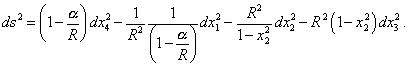(21)
 The matrix related to this metric has the form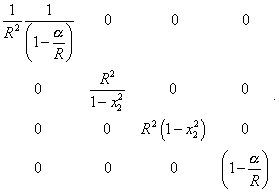(22)
 We can easily see from (22) that even in case of local field, when R = const, (22) is not reduced to (20). This means, the principle of light speed constancy is invalid even in a local reference frame, with all consequences for SR. The record of metric (21) in the initial rectangular frame will be even more complex and more obvious in its discrepancy to the requirements of locality which Einstein wrote by his own hand. If we add the evidence by Einstein who recognised the presence of material properties of space (which we gave in the item 2 of our paper and above), we will clearly see, how Einstein denied the void space as an inalienable condition of light speed constancy in all frames. And even what we give here is not and cannot be complete. Speaking of Einstein denying his postulates of SR, we have to give the proof that he denied also the principle of equivalence of physical laws in inertial and non-inertial reference frames, as these are related issues. Actually, as we showed above, Einstein, passing from SR in which exceptionally inertial frames are considered to GR in which non-inertial frames are considered, denied the postulate of constant speed of light. At the same time light is known to be a physical phenomenon and its propagation is determined by physical laws. If according to Einstein, the physical laws in inertial and non-inertial frames were equivalent, the light speed has to remain constant both in inertial and non-inertial frames. As well as Minkowski’s metric has to remain irrespectively of, whether the frame is inertial or not. But if the basic for the relativistic conception regularity of light propagation was violated, it appears senseless even to speak of equivalence of physical laws in inertial and non-inertial frames. It remains only to add to the said that proofs which we gave in this Supplement are not the only. There are very many evidences, as SR and GR always were fighting with insolvable discrepancies that arose again and again in the underpinning of these theories. This required either to deny the theory or to do what relativists did - they partially denied their initial views, vigilantly retaining only two basic items: invariance of 4-metric and validity of Lorentz transformations - namely the items that caused discrepancies. Such tactics permanently led to the fact that the introduced changes not only did not lift the discrepancies but drove them deeper and deeper into the problem, creating new and new discrepancies and mistakes. Basically, even what we showed here, doing not mentioning our previous studies on the Absolute problem [21, p. 308], Doppler effect [22, p. 308], astronomical aberration [23, p. 308], is quite enough to see the full bankruptcy of this trend, which, besides, gives the impassable modelling equations. Exceptionally because relativists do not squeamish using any ways for the sake to retain their conception, it still is in power, closing for the scientists really promising and resulting trends.

Contents / 1 / 2 / 3 / 4 / 5 / 6 / 7 / 8 / 9 / 10 / Paper